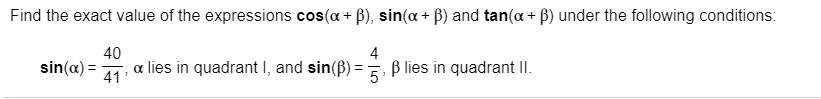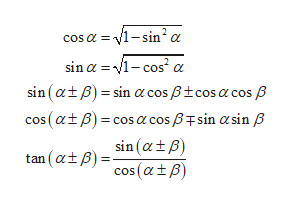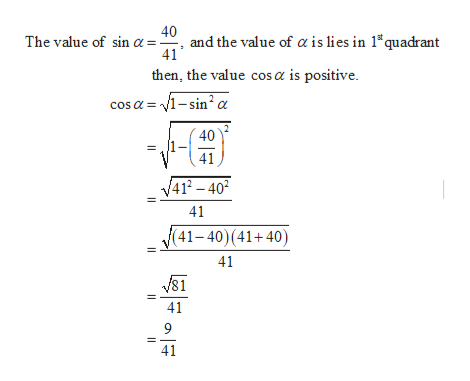# Find the exact value of the expressions cos(a B), sin(a + ) and tan(a B) under the following conditions:40lies in quadrant I, and sin(B) B lies in quadrant lI4sin(a)

Question
169 viewshelp_outlineImage TranscriptioncloseFind the exact value of the expressions cos(a B), sin(a + ) and tan(a B) under the following conditions: 40 lies in quadrant I, and sin(B) B lies in quadrant lI 4 sin(a) fullscreen
check_circle

star
star
star
star
star
1 Rating
Step 1

To calculate the value of the expression cos(α+β), sin(α+β) and tan(α+β) under the provided condition which is as sinα=40/41, α lies in first quadrant, and sinβ=4/5, β lies in second quadrant.

Step 2

Some formula of trigonometric ratio which is used here is shown below,help_outlineImage Transcriptionclosecosa 1-sin2 a sin a 1-cos sin (at )sin acos Btcos a cos cos (at) cos a cosBsin asin B sin (atB) tan (tp)cos(atB) fullscreen
Step 3

Now, first find the value of cos α and cos β. The value of cos &...help_outlineImage Transcriptionclose40 and the value of a is lies in 1 quadrant 41 The value of sin a then, the value cos a is positive cos a 1-sin2 2 40 41 4 40 41 41-40)(41+40) 41 41 9 41 fullscreen

### Want to see the full answer?

See Solution

#### Want to see this answer and more?

Solutions are written by subject experts who are available 24/7. Questions are typically answered within 1 hour.*

See Solution
*Response times may vary by subject and question.
Tagged in

### Trigonometric Ratios